# Other articles

1. Published: Fri 07 September 2018

# Finding Trade and Exchange Possibilities with Prolog

A friend recently had an interesting problem for me: There's a game for mobile phones where you have to exchange or transmute wares into each other, in order to create some strange supply chain.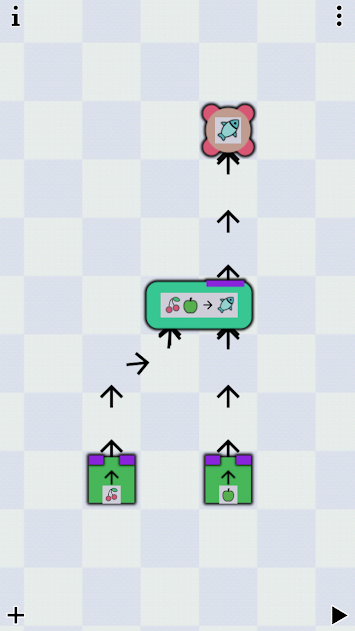Example of an earlier level.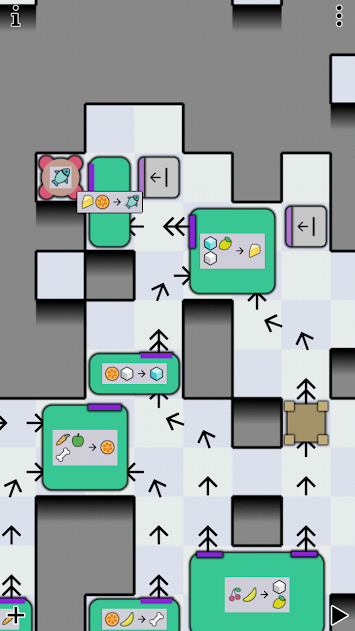A very complex later example.

The game is bleentoro, image copyright by them.

In this example (an earlier level) the goal is to create fish. We can transmute or exchange (I will only write about exchange from now on) an apple and a pair of cherries for a fish. Apple and cherry we have, or are created from thin air, in the context of the game. As you can see in the other example, this can get very complex.

The question is, given some input we have, in what order do we have to use the stations for exchange to create some output. How do we best model this problem?

## Modeling the Problem

From a previous article we already know that we can encode numbers in Prolog terms using Peano numbers. Using these we can match terms that represent at least or exactly some number. Exactly 3 would be s(s(s(z))) while at least 3 would be s(s(s(X))) with the variable X representing the rest of the term for some $$n$$ so that $$3 + x = n$$.

Let's start with a small example: we have an aunt that gives us apples. We have bob who is willing to exchange an apple for two berries. And we have a cousin who will bake a cake if we give them two apples and five berries.

Let's formalize our rules in some way:

a: / -> 1A
b: A -> 2B
c: 2A, 5B -> 1C


We model this as some kind of inventory(A, B, C) with some slots for Peano encoded numbers. Meaning that inventory(s(z), z, s(C)) means an inventory of exactly one apple, zero berries, and at least one cake. Lucky us.

Let's define our possible exchanges as Prolog facts with exchange(Kind, Pre, Post) meaning we use the exchange method Kind and we get from inventory state Pre to inventory state Post. If we lose an item, we have it in Pre but don't in Post. If we gain one, it's the other way around. Of course, the rest of our inventory should stay the same.

exchange(a, inventory(A, B, C), inventory(s(A), B, C)).
exchange(b, inventory(s(A),B,C), inventory(A, s(s(B)), C)).
exchange(c, inventory(s(s(A)), s(s(s(s(s(B))))), C), inventory(A, B, s(C))).


As we can see, we can encode basically any numeric condition that uses only "just exactly" and "at least", as well as addition and subtraction and "setting" fields to specific values. If we have to give at least three apples, but all of them, to get a cake, the rule would be exchange(c, inventory(s(s(s(_))), B, C), inventory(z, B, s(C)))..

## Programming the Solver

We want to create a predicate trades(Start, Goal, Path) where we give a Start inventory and a Goal inventory and want to get a Path of exchanges. The most obvious approach is the following:

trades(Goal, Goal, []).


So we're done when our Goal is reached. And if not, we have to try some exchange. We try it and we quickly learn that...

?- trades(inventory(z, z, z), inventory(_, _, s(z)), Path).
ERROR: Out of local stack


... it doesn't work.

The problem is, that calling the exchange predicate in the recursive call always tries the first rule first and since that basically always works, we get into an infinite loop. Let's reorder the rules and put the generating rule first.

?- trades(inventory(z, z, z), inventory(_, _, s(z)), Path).
ERROR: Out of local stack


It still doesn't work. Let's look at the trace.

[trace]  ?- trades(inventory(z, z, z), inventory(_, _, s(z)), Path).
Call: (8) trades(inventory(z, z, z), inventory(_700, _702, s(z)), _712) ? creep
Call: (9) exchange(_960, inventory(z, z, z), _984) ? creep
Exit: (9) exchange(a, inventory(z, z, z), inventory(s(z), z, z)) ? creep
Call: (9) trades(inventory(s(z), z, z), inventory(_700, _702, s(z)), _962) ? creep
Call: (10) exchange(_978, inventory(s(z), z, z), _1002) ? creep
Exit: (10) exchange(b, inventory(s(z), z, z), inventory(z, s(s(z)), z)) ? creep
Call: (10) trades(inventory(z, s(s(z)), z), inventory(_700, _702, s(z)), _980) ? creep
Call: (11) exchange(_1000, inventory(z, s(s(z)), z), _1024) ? creep
Exit: (11) exchange(a, inventory(z, s(s(z)), z), inventory(s(z), s(s(z)), z)) ? creep
Call: (11) trades(inventory(s(z), s(s(z)), z), inventory(_700, _702, s(z)), _1002) ? creep
Call: (12) exchange(_1018, inventory(s(z), s(s(z)), z), _1042) ? creep
Exit: (12) exchange(b, inventory(s(z), s(s(z)), z), inventory(z, s(s(s(s(z)))), z)) ? creep
Call: (12) trades(inventory(z, s(s(s(s(z)))), z), inventory(_700, _702, s(z)), _1020) ? creep
Call: (13) exchange(_1040, inventory(z, s(s(s(s(z)))), z), _1064) ? creep
Exit: (13) exchange(a, inventory(z, s(s(s(s(z)))), z), inventory(s(z), s(s(s(s(z)))), z)) ? creep
Call: (13) trades(inventory(s(z), s(s(s(s(z)))), z), inventory(_700, _702, s(z)), _1042) ? creep
Call: (14) exchange(_1058, inventory(s(z), s(s(s(s(z)))), z), _1082) ? creep
Exit: (14) exchange(b, inventory(s(z), s(s(s(s(z)))), z), inventory(z, s(s(s(s(s(s(z)))))), z)) ? creep
Call: (14) trades(inventory(z, s(s(s(s(s(s(z)))))), z), inventory(_700, _702, s(z)), _1060) ? creep
Call: (15) exchange(_1080, inventory(z, s(s(s(s(s(s(z)))))), z), _1104) ? creep
Exit: (15) exchange(a, inventory(z, s(s(s(s(s(s(z)))))), z), inventory(s(z), s(s(s(s(s(s(z)))))), z)) ? creep
Call: (15) trades(inventory(s(z), s(s(s(s(s(s(z)))))), z), inventory(_700, _702, s(z)), _1082) ? creep
Call: (16) exchange(_1098, inventory(s(z), s(s(s(s(s(s(z)))))), z), _1122) ? creep


We're still running into an infinite loop since we're just getting apples and trading them for berries.

There's a trick if our solution is a list. If the length(List, Length) predicate is called with both predicates free (length(L, _) for example), we get lists of increasing length as solutions. So if we can bind our solution to lists of increasing length, we won't get infinite loops of apple-berry-exchanges without ever trying short solutions.

So our query now looks like this:

?- length(Path,_), trades(inventory(z, z, z), inventory(_, _, s(z)), Path).
Path = [a, b, a, b, a, b, a, a, c] ;
Path = [a, b, a, b, a, a, b, a, c] ;
Path = [a, b, a, b, a, a, a, b, c] ;
Path = [a, b, a, a, b, b, a, a, c] ;
Path = [a, b, a, a, b, a, b, a, c] ;
Path = [a, b, a, a, b, a, a, b, c] ;
Path = [a, b, a, a, a, b, b, a, c] ;
Path = [a, b, a, a, a, b, a, b, c] ;
Path = [a, b, a, a, a, a, b, b, c] ...


As it turns out, there are a few ways to do it, but nothing shorter than 9 trades. We can even look, whether we have anything left over after we got our cake:

?- length(Path,_), trades(inventory(z, z, z), inventory(A, B, s(z)), Path).
Path = [a, b, a, b, a, b, a, a, c],
A = z,
B = s(z) .


Yeah. As it turns out, we have a berry to spare.

## Conclusion

This is how you do it. And of course, you could simulate a shop with that, as one inventory slot could be the number of coins. If the inventory gets too large, you can split it into item types so you don't have to copy every unchanged item from the pre-condition to the post-condition. Then you can copy a term that might represent any number of items.

But beware: this algorithm (with Prolog's evaluation scheme) has not so good complexity. For a solution of length $$n$$ and $$m$$ rules it has a run time of $$O(m^n)$$. We create every possible solution list permutation but break early, if no rules are applicable.

Depending on our instantiation of Start and Goal, this algorithm doesn't terminate (if the system isn't terminating, the algorithm isn't either). Our system doesn't terminate as we can always apply a again and again. We're creating increasingly longer partial solutions which might turn out to be no solution at all. If there is no solution, you won't know. The algorithm just tries longer and longer lists trying to find a solution.

If you have any other conditions on your solution (some exchanges might be limited or necessary) you can put most of them after the trades call. Some conditions, like membership, can be put before.

Let's say we have a terminating system by taking away the a rule. If our Start is fully instantiated, then our algorithm terminates. Let's try another query and find out, with how many apples we need to start to get a cake:

?- trades(inventory(A, z, z), inventory(_, _, s(z)), Path).
ERROR: Out of local stack


Through the unification rules, it is possible that a rule creates terms on the left side of the rule. In this case, the algorithm tries to give us increasing amounts of berries to match that with the starting condition (only rule b is applied in reverse, always leaving us with one cake, which doesn't match the starting condition). There we need the length predicate.

?- length(Path,_), trades(inventory(A, z, z), inventory(_, _, s(z)), Path).
Path = [b, b, b, c],
A = s(s(s(s(s(_2334))))) .


We need at least five apples for a cake. But we knew that.

To recap:

• We saw again how useful it is, to encode the natural numbers in a term structure.
• A solver is easily written but the algorithm isn't fast.
• The rules can be applied in both directions because of Prolog's evaluation scheme. This leaves queries that would intuitively work divergent.
• By using the length predicate we can simulate breadth-first evaluation, while the default evaluation scheme is depth-first.

I think, there's a different approach using linear programming. I might try that in the future.

2. Published: Mon 27 August 2018

I love watching 8 Out Of 10 Cats Does Countdown. It's a british panel show where a few comedians solve word puzzles and mathematics puzzles. It's based on the game show Countdown that's been running since 1982.

And I also like the host Jimmy Carr very much.

## The Puzzles

The show consists of three different puzzles:

In the Letters round a team (or single contestant) picks 9 consonants and vowels from their respective piles. After the team chooses from one of both piles, the letter is revealed and put up on the board. They then choose again until 9 letters are on the board. After all letters are on the board, the teams have 30 seconds to form a word out of as many of the letters as possible. The team with the longest word scores that many points.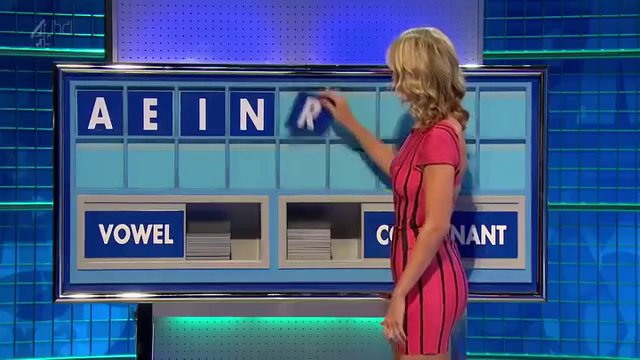Letters Round

In the Conundrum a list of letters that already spell out a funny group of words is given by the host and the contestants try to find a 9 letter anagram. The first team to buzzer in the correct answer gains 10 points. This is the final round of the game.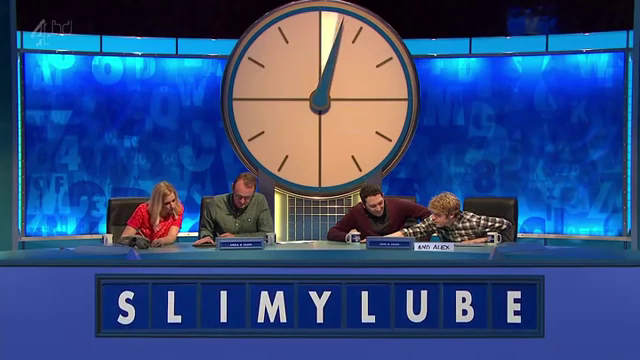Conundrum

In the Numbers round a team (or single contestant) picks 6 numbers from two piles. A random number is picked and the contestants have to create a mathematical formula out of the picked numbers that equal the target number. The specific rules follow.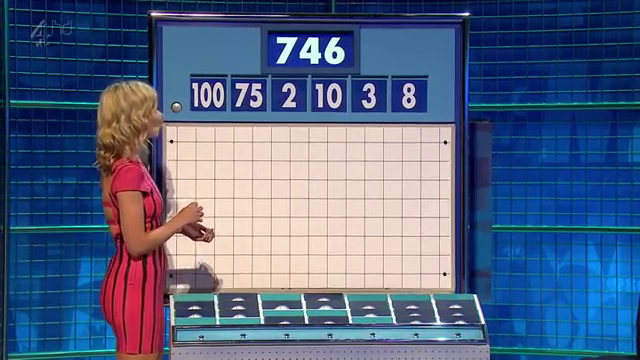Numbers Round

## Numbers Round

According to Wikipedia there are 6 columns of 4 numbers (4 rows). One column contains the numbers 25, 50, 75, and 100 and the other 5 columns contain the numbers 1 to 10 twice each. That's not exactly the same as on the show, as there appear to be 7 columns of 4 numbers but not every stack has all 4 number cards.

Contestants choose a total of 6 numbers from the "small" and "large" columns. The usual pick would be 2 to 3 "large" numbers and the rest small ones.

Then a random number between 100 und 999 is generated. The contestants try to create a mathematical formula that has the random number as the result. In the formulare only the following operations are allowed:

• Multiplication
• Subtraction (that does not result in a negative number)
• Division (that results in an integer)

The contestants do not have to use all the numbers to get the target number.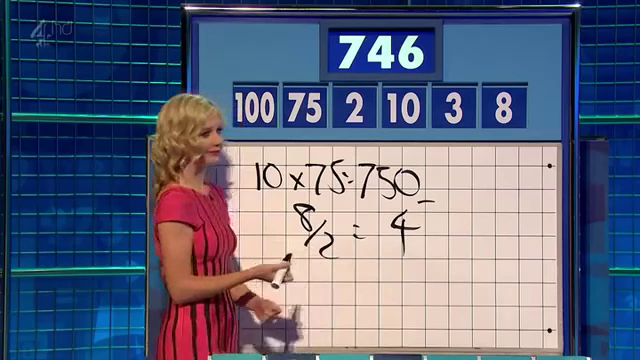Finished Numbers Round

Since I am really not good at this, I wrote a solver for the numbers game in Haskell.

## Solver

How do we go about this problem? First of all, we have to decide on a data structure. Let's start with a traditional term structure where a Term is a binary operation and two terms, or an integer value. An Operation is the function mapping two integers to a third, and a string to pretty-print the operation. The mapping is not total and might fail.

data Term = Op Operation Term Term | Val Integer
data Operation = Operation {fun :: (Integer -> Integer -> Maybe Integer), name :: String}

instance Show Term where
show (Val x) = show x
show (Op op l r) = "(" ++ (show l) ++ (name op) ++ show r ++ ")"


Now we have to define our operations and what they should do. Since we're using Maybe, and it's a monad, we can use monadic notation where it suits us:

operations = [  Operation (\x y -> return (x + y)) "+"
,  Operation (\x y -> return (x * y)) "*"
,  Operation (\x y -> guard (x > y) >> return (x - y)) "-"
,  Operation (\x y -> guard (x mod y == 0) >> return (x div y)) "/"
]
eval :: Term -> Maybe Integer
eval (Val v) = Just v
eval (Op o l r) = do l' <- eval l
r' <- eval r
(fun o) l' r'


As we can see, the preconditions to the subtraction and division are encoded using the guard function. If the function returns true, the whole operation returns Nothing, otherwise it returns Just the value.

Since Haskell uses lazy evaluation, we can define a list of all possible terms given a list of integers and filter for the ones that evaluate to the correct number.

As we create a Term we have to be careful to not use any of the numbers again. So we will split our numbers in two sets. One that can be used in the left Term of an Operation and the rest can be used in the right Term. We will create all terms using our definition. A Term is either the Value of one of the numbers for that subterm, or an operation with some numbers reserved for the left term and some numbers reserved for the right term.

terms :: [Integer] -> [Term]
terms nums =  do  n <- nums -- choose a number
[Val n] -- one solution
++
do  op <- operations -- choose an operation
(l, r) <- subset_split nums -- choose a split
guard $l /= [] guard$ r /= []
ls <- (terms l) -- choose a term for the left side
guard (eval ls /= Nothing)
rs <- (terms r) -- choose a term for the right side
guard (eval rs /= Nothing)
[Op op ls rs] -- one solution


For performance reasons we already evaluate the generated subterms in order to check whether the operations are valid. There are a lot of terms that can not be evaluated and we want to throw them out as early as possible (or not even generate them).

We haven't defined subset_split yet. The idea is, to just split the list of numbers in two groups in every possible way:

subsets :: [Integer] -> [[Integer]]
subsets []  = [[]]
subsets (x:xs) = subsets xs ++ map (x:) (subsets xs)

subset_split nums = do l <- subsets nums
return (l, nums \\ l)


Now all that remains is to generate all terms given a set of numbers, evaluate them, filter out the ones that don't have the correct value, and return the first one:

solve :: [Integer] -> Integer -> Maybe Term
solve nums target = let possible_solutions = map (\x -> (x, eval x)) (terms nums)
solutions = filter (\x -> (snd x) == (Just target)) possible_solutions
in listToMaybe $map fst solutions  And in our main we only read the list of numbers and the target as arguments and print the solution: main = do args <- getArgs let nums = (read (args !! 0))::[Integer] target = (read (args !! 1))::Integer print$ solve nums target


And there we go:

$./countdown "[100, 75, 2, 10, 3, 8]" 746 Just (8+(3+((75*(100-2))/10)))  Our solution is $$8+3+{{75*(100-2)} \over 10}$$ while the solution from the show was $$10*75 - {8 \over 2}$$. As you can see, we do not always get the easiest solution but we usually have a solution quite fast. This one took about a tenth of a second. The 30 seconds given to the contestant are usually quite enough. To make sure we had the "nicest" solution, we probably would need to generate all terms and sort them by size. The smallest one should be the nicest one. 3. Published: Sat 11 August 2018 # Lost in Localisation - Ordering a Taxi while on Holiday I was on holiday in England this summer. I arrived in Worthing by bus from London, had a full day there, and wanted to take the train the following day to Bristol. The bus route from my BnB to the station was inconvenient and expensive. Ordering a taxi was my next idea. I wanted to do that without human interaction the evening before. This is the story of how that did not work. I name the taxi company in this article. This is not to shame them. I hope they just fix the issues, which I believe present in many applications, because localisation is hard.Quite a Promise ## The Mobile App Before analyzing why ordering a taxicab through their website did not work, I wanted to try the mobile app. I was hopeful, considering their promise that I could "book a car in seconds and don't even need to ring us!". As it turns out, this app is not available through the German Google Play Store. This means, you won't find it when you search for it and if you have the correct URL, you can't install the app through the web interface on a device that is connected to the German Google Play Store (so all of mine). But this is not a problem since you can download any APK that is available from any app store through a third-party service like APKPure (unendorsed). So knowing the name of the app in the Play Store I did that and installed the app.That is the App You're presented with a registration screen where you have to enter your phone number to receive a confirmation SMS. My (international) phone number didn't take and I was not able to complete the process. We will later see why. Being unable to register via the app, I return to the web site.The website looks quite modern ## The Web SiteThe first part of the order form As you can see, to order a taxi you first have to enter where you want to be picked up and where you want to go. The system then calculates the distance between both points, estimates the cost, and then you can go on to the next step. In order to calculate the distance, the web site uses the Google Maps API to create a route between both points and calculates a cost. Here's the corresponding code from the site: journey['distance'] = response.routes.legs.distance; journey['duration'] = response.routes.legs.duration; journey['miles'] = ( journey['distance'].value * 0.000621371192 ); journey['price'] = CurrencyFormatted(2.90); journey['total'] = CurrencyFormatted( journey['price'] + 1.90 * ( journey['miles'] - 0.2 )); journey['start_address'] = response.routes.legs.start_address; journey['end_address'] = response.routes.legs.end_address;  This looks okay. It is to note that the site takes the addresses returned by google as the canonical start and end of the journey. So in principle, you could enter the name of a shop or restaurant as the target location of your taxicab ride and as long as google knows it, the taxi service is booked to the actual and correct address. Then there's another step of validation: Since the taxicab company only operates in Worthing in the south of England, they do not want to accept rides that are outside a specific area. They want to achieve this by parsing the post code from the canonical google response and compare it to a set of given post codes that correspond to the Worthing area: journey['start_postcode'] = journey['start_address'].match(/([A-Z]?\d(:? \d[A-Z]{2})?|[A-Z]\d{2}(:? \d[A-Z]{2})?|[A-Z]{2}\d(:? \d[A-Z]{2})?|[A-Z]{2}\d{2}(:? \d[A-Z]{2})?|[A-Z]\d[A-Z](:? \d[A-Z]{2})?|[A-Z]{2}\d[A-Z](:? \d[A-Z]{2})?),\s*UK$/). That's quite a large regular expression and I give you a visualisation powered by Regexper:

This does correspond in some fashion (but not exactly ) to the valid UK post codes followed by ", UK" and the end of the input.

I give you part of the google response for a ride between some random house and the train station and let you figure out why this step fails for me:

{ "routes" : [
{ "legs" : [
{
"distance" : {
"text" : "1,7 km",
"value" : 1654
},
"duration" : {
"text" : "5 Minuten",
"value" : 292
},
"end_address" : "Worthing BN11 1AA, Vereinigtes Königreich",
"start_address" : "Harrow Rd, Worthing BN11 4RB, Vereinigtes Königreich"
}
]}
]}


Google does return the post code correctly, but since my browser is set to German, Google's response is in German as well. The regular expression only matches strings ending in "UK", while my German response ends in "Vereinigtes Königreich" ("United Kingdom" in German).

The fix seems easy enough. Just set the browser language to UK English and do the whole thing again and there we go:Success?Okay, good. Let's book the taxi.

Now we just have to enter our name, email address, and phone number and we're good and finally can go by taxi wherever we want to go (within the greater Worthing area). As I enter my phone number, starting with +49 or 0049 for Germany, followed by three digits for the service provider , and seven or eight digits for the user, it dawns on me that it might not be that easy (as if it had been up to this point) and I take another glance at the code:

function validateTelephone(telephone){
var re = /^(?:\W*\d){11}\W*$/; return re.test(telephone); }  Oh oh. Okay. Yeah. About that... My phone number does not fit that scheme (while 00000000000 does). If I get any confirmation code I need to respond to, or they want to call back for some additional questions, they won't be able to reach me. This is probably also the reason why the app did not work and why I couldn't receive an SMS with a confirmation code. I won't be able to order a taxi online. To recap the two main reasons why: • Because my browser is set to something else than English, the website can not verify that the cab journey takes place within the serviced area. Solution: Google provides GPS coordinates for the legs of the journey. One can easily check whether they are contained within a rectangle or convex polygon. • Because I have an international phone number, I can't interact with the parts of the process that depend on my cellphone. Solution: Allow for international phone numbers or make the process not depend on them. In the end I called them by phone, which took a whopping 25 seconds and the taxi arrived on time to bring me to the train station.   According to Wikipedia, the UK government has provided a regular expression that can be used for the purpose of validation: ^([Gg][Ii][Rr] 0[Aa]{2})|((([A-Za-z][0-9]{1,2})|(([A-Za-z][A-Ha-hJ-Yj-y][0-9]{1,2})|(([A-Za-z][0-9][A-Za-z])|([A-Za-z][A-Ha-hJ-Yj-y][0-9]?[A-Za-z])))) [0-9][A-Za-z]{2})$
  It's a little bit more complicated but sufficient for this story.

4. Published: Wed 18 July 2018

# Prolog Programs, Order of Evaluation, and Functional Equivalents

While teaching Prolog this semester I had a few thoughts about Prolog programs and their equivalent programs in a functional programming language like Haskell.

## The Peano Naturals

The Peano numbers are a unary number system for positive natural numbers where the zero is represented by a Z (or 0, or any atom - it doesn't matter) and every successor of a natural number N is the function S applied to N. We would therefore represent the natural number 3 with the term S(S(S(Z))). To look at it differently: in the Peano axioms, the naturals are defined as the set that contains zero and a closure over the successor function. In haskell we would define the type of the naturals like this:

data N = Z | S N


In Prolog we don't need a type definition for the naturals, as terms are generated without types. Now we want a predicate that checks whether our input is a natural number as we've defined it. The idea is that the term is a natural, iff either the term is a Z or it has an S as its root and its parameter is a natural. In Prolog we would write it intuitively like this:

naturals(z).
naturals(s(X)) :- naturals(X).


And indeed this checks whether our input is a natural number. The query naturals(s(s(z))). returns true while naturals(foo). does not. Writing down this program in Haskell is not very useful since the type system does not allow us to have anything else but a natural as the function parameter but let's pretend:

naturals v = case v of
S x -> naturals x
Z -> True
_ -> False


And of course, calling naturals $S$ S Z in haskell has the correct type and returns true. But this haskell program only corresponds to the case where we actually call the Prolog predicate with a bound parameter. We could call the prolog predicate with a free binding pattern like naturals(X). and get bindings for X for every solution, so every natural number. The corresponding Haskell programm would be:

naturals_f  = [ Z ] ++ map S naturals_f


This might need some explaining: The list corresponds to some notion of non-determinism. In Prolog the non-determinism is baked into the language due to backtracking from failed predicate evaluations and us asking for more answers. The Prolog program essentially says: Z is a solution. Everything with an S is a solution, if the "content" of the S is a solution as well. That's the same for our haskell programm. In our list of solutions we know that Z is in it and for every solution we have, adding an S leads to another one. And evaluating naturals_f starts to calculate the infinite list of natural numbers.

Now consider the following Prolog program where we switched the order of the clauses:

naturals(s(X)) :- naturals(X).
naturals(z).


This still works nicely for the bound case where we call naturals(s(s(z)). But if we now call naturals(X) we quickly get ERROR: Out of local stack. The natural intuition might be that the order of clauses does not matter but it does in this case. Writing down the equivalent Haskell programm we are able to see why:

naturals_f  = map S naturals_f ++ [ Z ]


We have the recursion before we have any solution. Well, the solution is easy: just write the simple case in the first line and the recursive case in the second. But let's consider another problem.

## Repeated Printing

Consider the problem of repeatedly printing a character:

repeat(0, _).
repeat(N, C) :- write(C), NN is N - 1, repeat(NN, C).


This looks very correct and is very wrong.

?- repeat(5, f).
fffff
true ;
ffffffffffffffffffffffffffffffffffffffffffffffffffffffffffffffffffffffffffffffffffffffffffffffffffffffffffffffffffffffffffffffff...


Now we see why it's nice to represent the naturals als Peano numbers. After hitting 0 there's a choice point left and asking for another solution leads to the negatives and printing our character in an infinite loop. So let's augment the second rule to prevent us from going into the negatives.

repeat(0, _).
repeat(N, C) :- N > 0, write(C), NN is N - 1, repeat(NN, C).
% query:
?- repeat(5, f).
fffff
true ;
false.


This looks better but we still have a choice point left over which might be not so nice. The problem is that the matched patterns are not disjoint and after the end of the recursion there's still a pattern left over that fits. We can't encode that N should be larger than 0 in the head of the rule. Remember that we can match naturals larger than 0 (or any other number) in the rule head using Peano numbers - that's why they are so cool.

But how do we fix our problem with the left-over choice point? There are two ways we can do it. We can either introduce a cut or reorder the rules.

% Variant 1a: The order doesn't matter but we introduce a cut
repeat(0, _) :- !.
repeat(N, C) :- N > 0, write(C), NN is N - 1, repeat(NN, C).

% Variant 1b: If we promise to be good and only call repeat with a positive numeric argument, we can even leave out the range check
repeat(0, _) :- !.
repeat(N, C) :- write(C), NN is N - 1, repeat(NN, C).

% Variant 2: The order does matter
repeat(N, C) :- N > 0, write(C), NN is N - 1, repeat(NN, C).
repeat(0, _).


I personally think that the variant without the cut is nicer. So for terminating rules, it might be better to have the "smaller" rule last as to not leave choice-points hanging around. Of course, this only works if the matched terms in the rules are proper subsets of each other so that we actually can order the rules in that fashion. In general, that might not be possible.

## Conclusion

• The order of rules can (in theory) not affect termination. But if our rules are already non-terminating for our query, the order and timeliness of results does matter. Then order does matter.
• Disjoint rule heads are important to not have left-over choice points.
• If rules are terminating but the rule heads are not disjoint, they still should be ordered carefully.
• Implicit conditions (N > 0) can lead to non-obvious non-termination if they are not made explicit.
• By encoding data properties in the term structure we can make some implicit conditions explicit and make rule-heads disjoint.
• Having numerical conditions in rule-heads would be really nice. Maybe some Prolog implementation in the future allows for that.

Page 1 / 6 »